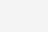# 6 其它区别

sizeof引用的时候是对象的大小，sizeof指针是指针本身的大小；

# 7 代码说明

int a,b,*p,&r=a; //正确

r=3; //正确：等价于a=3

int &rr; //出错：引用必须初始化

p=&a; //正确：p中存储a的地址，即p指向a

*p=4; //正确：p中存的是a的地址，对a所对应的存储空间存入值4

p=&b; //正确：p可以多次赋值，p存储b的地址

# 通俗化说明

int a = 0;

int b = 1;

int *point = NULL;

point = &a; // 在某个时刻，指针可以指向a

point = &b; // 换个时刻，指针可以指向b

int xiaoming = 1;

int &refence_mingming = xiaoming;

int xiaoan = 2;

refence_mingming = xiaoan; // error,引用不能换了

# 从对象的角度理解指针

C++primer中对 对象的定义：对象是指一块能存储数据并具有某种类型的内存空间

# 引用的主要功能是传递函数的参数和返回值。

C++语言中，函数的参数和返回值的传递方式有三种：值传递、 指针传递和引用传递。

void Func1(int x) {

x = x + 10;

}

int n = 0;

Func1(n);

cout << “n = ” << n << endl; // n = 0

void Func2(int *x) {

(* x) = (* x) + 10;

}

int n = 0;

Func2(&n);

cout << “n = ” << n << endl; // n = 10

void Func3(int &x) {

x = x + 10;

}

int n = 0;

Func3(n);

cout << “n = ” << n << endl; // n = 10

－End－(0)

### 相关推荐

• #### 单板透视（单板透视会封号吗）

单板透视（单板透视会封号吗）

• #### mcafee企业版8.8（mcafee手机版）

mcafee企业版8.8（mcafee手机版）

r2v教程（r2s教程）

• #### 华硕人脸识别（华硕win10人脸识别设置不了）

华硕人脸识别（华硕win10人脸识别设置不了）

• #### skiller3.5（skills for success 3）

skiller3.5（skills for success 3）

• #### timestampdiff（oracle中timestamp）

timestampdiff（oracle中timestamp）

• #### node.js安装（为什么nodejs不适合大型项目）

node.js安装（为什么nodejs不适合大型项目）

• #### 好帮手软件（作业好帮手下载）

好帮手软件（作业好帮手下载）